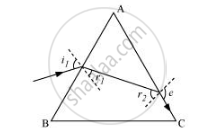# At what angle should a ray of light be incident on the face of a prism of refracting angle 60° so that it just suffers total internal reflection at the other face? - Physics

Numerical

At what angle should a ray of light be incident on the face of a prism of refracting angle 60° so that it just suffers total internal reflection at the other face? The refractive index of the material of the prism is 1.524.

#### Solution

The incident, refracted, and emergent rays associated with a glass prism ABC are shown in the given figure.Angle of prism, ∠A = 60°

Refractive index of the prism, µ = 1.524

i1 = Incident angle

r1 = Refracted angle

r2 = Angle of incidence at the face AC

e = Emergent angle = 90°

According to Snell’s law, for face AC, we can have:

(sin "e")/(sin "r"_2) = µ

sin "r"_2 = 1/µ xx sin 90°

= 1/1.524

= 0.6562

∴ r2 = sin−1 0.6562 ≈ 41°

It is clear from the figure that angle A = r1 + r2

∴ r1 = A − r2 = 60 − 41 = 19°

According to Snell’s law, we have the relation:

µ = (sin "i"_1)/(sin "r"_1)

sin "i"_1 = µ sin "r"_1

= 1.524 × sin 19°

= 0.496

∴ i1 = 29.75°

Hence, the angle of incidence is 29.75°.

Concept: Refraction Through a Prism
Is there an error in this question or solution?

#### APPEARS IN

NCERT Physics Part 1 and 2 Class 12
Chapter 9 Ray Optics and Optical Instruments
Exercise | Q 9.22 | Page 346
NCERT Class 12 Physics Textbook
Chapter 9 Ray Optics and Optical Instruments
Exercise | Q 22 | Page 348
Share